# BitAnd

## BitAnd(x, I)

Returns the bitwise AND of the integer portions of an array of numbers. Each bit in the result is on only if it is on in every integer in «x» along index «I». «I» can be omitted when taking the bitwise AND across the Implicit dimension.

Each integer may have up to 64 bits.

## Examples

To find the bitwise And of three numbers, the numbers can be listed in brackets (the implicit index) and the index parameter omitted.

BitAnd([23, 29, 25]) → 17

This is more obvious when we write these same numbers in binary notation:

BitAnd([0b10111, 0b11101, 0b11001]) → 17 { note: 17 = 0b10001 }

Suppose x is the following 2-D array of integers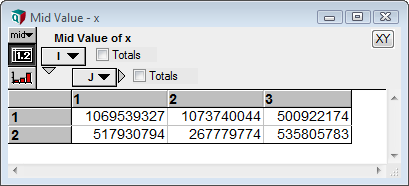which is shown here in binary notation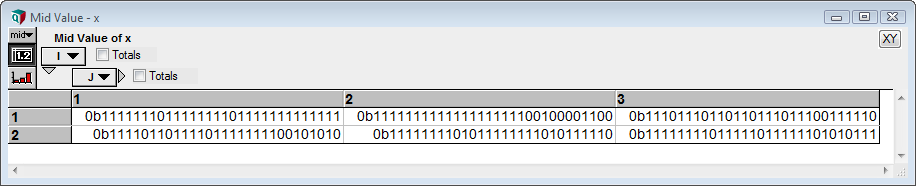then

BitAnd(x, I) →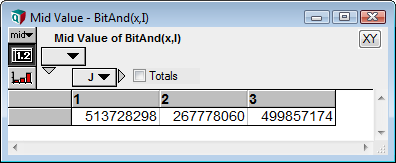=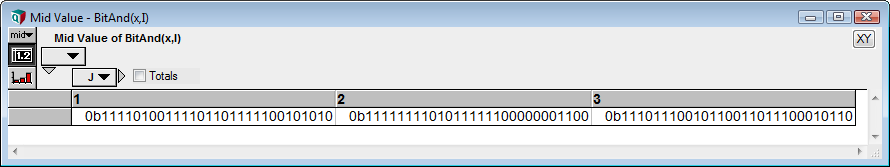BitAnd(x, J) →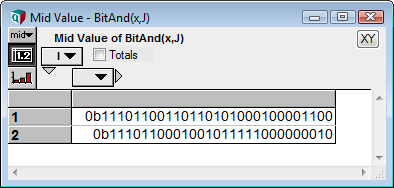## History

Introduced in Analytica 5.0.